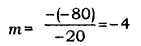# A student wants to project the image of a candle flame on a screen 80 cm

A student wants to project the image of a candle flame on a screen 80 cm in front of a mirror by keeping the candle flame at a distance of 20 cm from its pole.
(i) Which type of mirror should the student use?
(ii) Find the magnification of the image produced.
(iii) Find the distance between the object and its image.

(i) A real image can be projected on a screen using a concave mirror. In the given case, the student should use a concave mirror.

(ii) Magnification (m) is given by m = -v/u.
Here, u =Distance of the object v = Distance of the image(iii) The object and the image are on the same side of the mirror.
Distance of the object from the lens = 20 cm
Distance of the image from the lens = 80 cm
Thus,
Distance between the object and the image = 80 cm - 20 cm = 60 cm